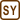## Classical view on the hydrogen atom

The total energy of the electron in the ground state is −13.6 eV. We consider it as a classical particle which moves around proton on a circular trajectory. Determine its radius, potential energy, kinetic energy and the velocity of the electron.

• #### Notation

 E1 = −13.6 eV Energy of the electron in the hydrogen atom in ground state r = ? (m) Radius of the trajectory of the electron (so called Bohr’s radius) Ek = ? (eV) Kinetic energy of the electron Ep = ? (eV) Potential energy of the electron v = ? (m.s-1) Velocity of the electron

From tables:

 c = 1.6·10−19 C Electron charge me = 9.1·10−31 kg Electron mass ε0 = 8.8·10−12 Fm−1 Vacuum permittivity
• #### Hint

We assume that the electron moves around the nuclei (proton) in a circle. This means that the electric force between them takes part as centripetal force.

The total energy of the electron consists of the kinetic energy and electrostatic potential energy which is negative because the electron and proton attract each other.

Therefore we obtain two equations with 2 unknowns – velocity of the electron and the electron’s distance from the nuclei.

• #### Analysis

The total energy of the electron consists of the kinetic energy and potential energy. Potential energy is electrostatic potential energy given by the effect of the electric forces between the electron and the hydrogen nuclei (proton). This energy depends on the charges of both particles and their distance (radius of the electron’s trajectory); furthermore, because both particles attract each other, the energy is negative.

The kinetic energy depends on the velocity. But the velocity of the electron needs to satisfy the fact that electric force from the nuclei is equal to centripetal force that holds it on its trajectory. From these two forces and the distance we can determine the velocity of the electron.

After these calculation the only unknown value in order to determine total energy is the radius of the trajectory that we will calculate. From substitution with radius we can also obtain other characteristic values for the electron.

• #### Solution

The total energy E is given by the sum of the kinetic Ek and potential Ep energies where potential energy is the electrostatic potential energy:

$E=E_{\mathrm{k}}+E_{\mathrm{p}}=\frac{1}{2}m_{\mathrm{e}}v^2-k\frac{e^2}{r} .$

The velocity of the electron must satisfy the means of the electric force Fe being the centripetal force Fc:

$F_{\mathrm{e}}=F_{\mathrm{c}} ,$ $k\frac{e^2}{r}=m_{\mathrm{e}}\frac{v^2}{r} .$

We can express the velocity v

$v^2=k\frac{e^2}{m_{\mathrm{e}}r}$

and substitute it into the formula for the total energy E

$E=\frac{1}{2}m_{\mathrm{e}}\frac{ke^2}{m_{\mathrm{e}}r}-k\frac{e^2}{r}=-\frac{ke^2}{2r} .$

We can determine the keen radius r:

$r=-\frac{ke^2}{2E}=-\frac{e^2}{8\pi\epsilon_0 E_1}$

and we substitute known values and the total energy E will be substituted by the energy of the electron in the ground state E1 which we will convert to jouls:

$r=-\frac{\left(1.6{\cdot}10^{-19}\right)^2}{8\pi\cdot8.8{\cdot}10^{-12}\cdot\left(-13.6{\cdot}1.6{\cdot}10^{-19}\right)}\,\mathrm{m}=5.3{\cdot}10^{-11}\,\mathrm{m} .$

The potential energy Ep of the electron is

$E_{\mathrm{p}}=-k\frac{e^2}{r}=-\frac{ke^2}{-\frac{ke^2}{2E_1}}=2E_1=-27.2\,\mathrm{eV} .$

We will determine the kinetic energy Ek of the electron from the formula for total energy

$E_1=E_{\mathrm{k}}+E_{\mathrm{p}}$ $E_{\mathrm{k}}=E_1-E_{\mathrm{p}}=E_1-2E_1=-E_1=13.6\,\mathrm{eV}$

and the velocity from the formula for kinetic energy

$E_{\mathrm{k}}=\frac{1}{2}m_{\mathrm{e}}v^2\,\Rightarrow\,v=\sqrt{\frac{2E_{\mathrm{k}}}{m_{\mathrm{e}}}}=\sqrt{\frac{-2E_1}{m_{\mathrm{e}}}}$ $v=\sqrt{\frac{-2\cdot\left(-13.6{\cdot}1.6{\cdot}10^{-19}\right)}{9.1{\cdot}10^{-31}}}\,\mathrm{m\,s^{-1}}\,\dot{=}\,2.2{\cdot}10^6\,\mathrm{m\,s^{-1}} .$